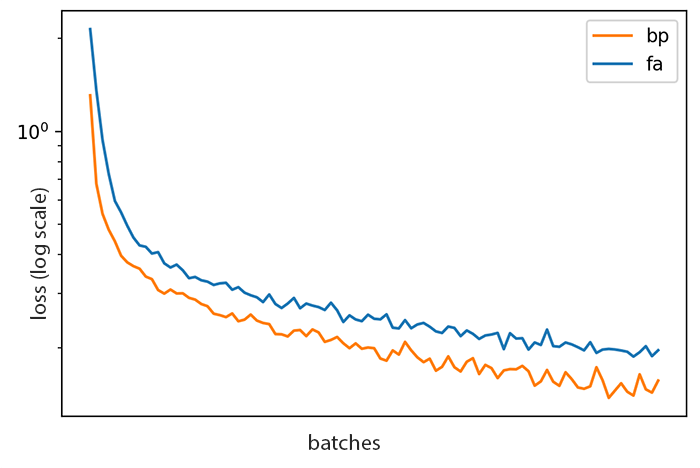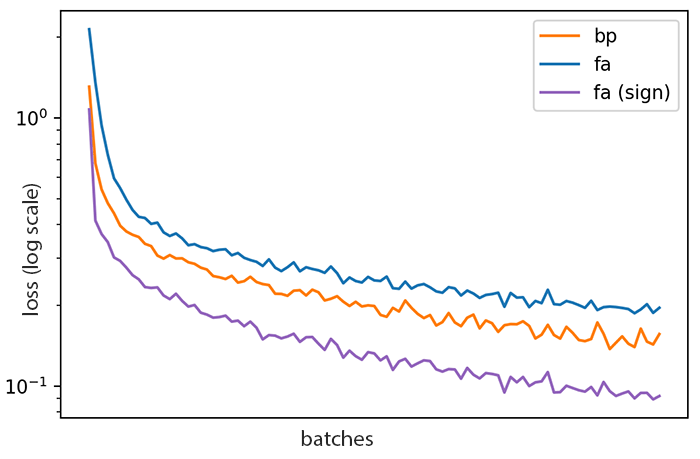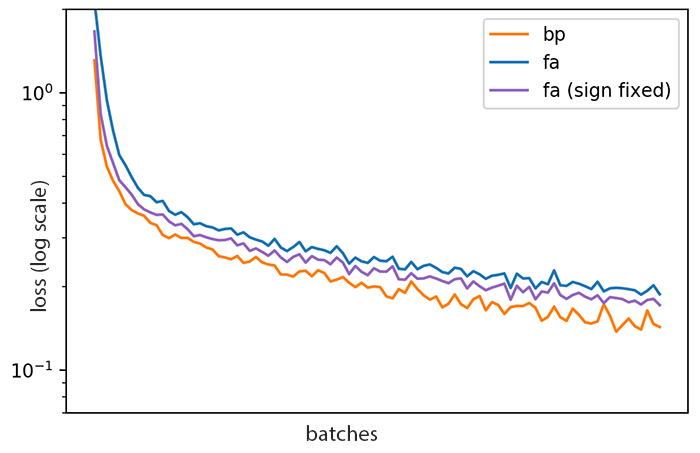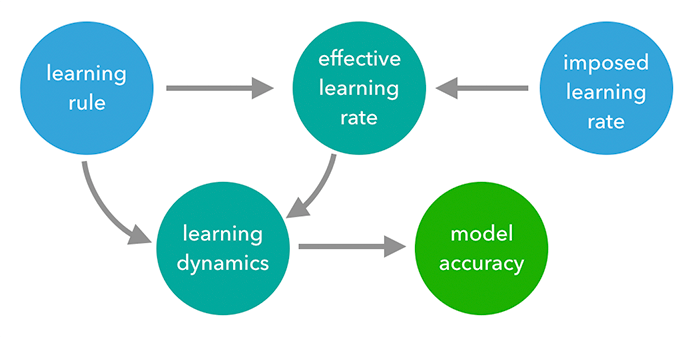SOAP (Second Order Activity Propogation) is a package for experimenting with feedback alignment and activity propogation in PyTorch. It formed my project for the 3-week IBRO-Simons Computational Neuroscience Summer School in 2019.

I’d like to share my journey over the few days I spent on this project, because it took me far afield and led to some strong opinions (always a good outcome).

### The weight transport problem

TODO: Describe it

For more information on Feedback Alignment, see Lillicrap’s original paper1.

### Vanilla feedback alignment

To start, I wanted to get feedback alignment training an MLP on MNIST. Using a modern framework like pytorch this turns out to be pretty easy to do, though slightly more verbose than I would expect. We can write a FeedbackAlignmentLinear layer that behaves like the standard Linear for the forward pass, but uses a custom, random B-matrix to backpropogate gradients:

class FALinearFunction(Function):

@staticmethod
def forward(ctx, data_in, weight, bias, b_matrix):
ctx.save_for_backward(data_in, weight, bias, b_matrix)
return data_in.mm(weight.t()) + bias.unsqueeze(0).expand_as(data_in)

@staticmethod
def backward(ctx, grad_out):
data_in, weight, bias, b_matrix = ctx.saved_tensors
return grad_out.mm(b_matrix), grad_out.t().mm(data_in), grad_out.sum(0), None

class FALinear(nn.Module):
def __init__(self, num_in, num_out):
super().__init__()
self.weight = nn.Parameter(torch.Tensor(num_out, num_in))
self.bias = nn.Parameter(torch.Tensor(num_out))
b_matrix = torch.zeros(num_out, num_in)
self.register_buffer('b_matrix', b_matrix)
init.xavier_normal_(b_matrix)
init.xavier_normal_(self.weight)
init.uniform_(self.bias, 0, 0)


This layer does indeed train on MNIST, though more slowly than standard backpropogation:Loss curves for MLP on MNISTFeedback alignment (FA) vs ordinary backpropogation (BP)

Although the two curves above look close, drawing a horizontal line through both curves shows that FA takes roughly twice as long to reach a given loss as BP — at least on this particular run.

Can we improve on this?

### Improving on FA

Muskovitz et al showed2 that we can improve FA by “breaking the rules” a bit: instead of allowing no weight transport, we can allow a little bit of information to periodically leak into the B matrix.

Let’s start with one bit of information leaking into $B$ from $W$, per neuron: merely the sign of the transpose of the weight matrix, updated only every $T$ examples rather than after every example.

We can write this as $B_t \leftarrow \operatorname{sign}(W_t^T)$ where $t \in \{T, 2T, 3T, \ldots\}$.

We indeed get quite a jump in performance. This sign-sychronized feedback alignment appears to outperform backprop, which is quite surpising!Sign-tracking feedback alignment v.s. the others

Unfortunately this single run is misleading. There is a subtle effect here, having nothing nothing to do with feedback alignment, which is actually responsible for this increased performance. It is related to learning rates: for this run we happened to have picked an SGD learning rate that was lower than necessary. Our update rule for $B$ has the effect of amplifying the (pseudo)gradient signal, an effect that more than compensates for the inaccuracy of using an incorrect gradient.

We can remove this effect by modifying the update rule to $B_t \leftarrow |B_0| \operatorname{sign}(W_t^T)$, so that the magnitude of the original feedback weights is preserved. Sure enough, the performance gain largely disappears, and this form of FA is still worse than ordinary back-propagation:Sign-tracking feedback alignment v.s. the others

The following causal diagram captures the confounding effect that the naive sign-change learning rule has on the model performance by modifying the average effective learning rate.Effective learning rate: confounding factor

1. Moskovitz, Theodore H., Ashok Litwin-Kumar, and L. F. Abbott. “Feedback Alignment in Deep Convolutional Networks.” ArXiv:1812.06488 [Cs, Stat], June 10, 2019. http://arxiv.org/abs/1812.06488. ↩︎

2. Lillicrap, Timothy P., Daniel Cownden, Douglas B. Tweed, and Colin J. Akerman. “Random Synaptic Feedback Weights Support Error Backpropagation for Deep Learning.” Nature Communications 7 (November 8, 2016): 13276. https://doi.org/10.1038/ncomms13276. ↩︎